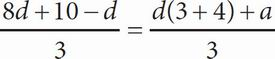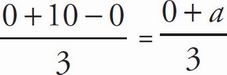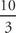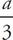# SAT Math Multiple Choice Question 171: Answer and Explanation

### Test Information

Question: 171

6.If the equation above has infinitely many solutions for d, what is the value of a ?

• A. -10
• B. 10
• C. 15
• D. 20

B The question states that there are infinitely many solutions to the equation. That means any real number should work for d. Plug in an easy number like 0 for every d in the equation to get. Simplify the equation to=, so a = 10, which is (B).